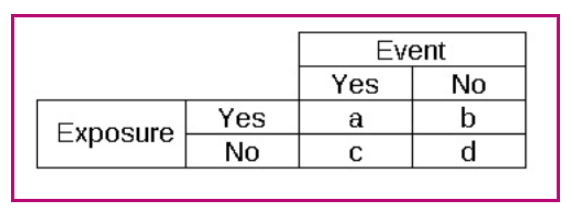Risk in statistical terms refers simply to the probability that an event will occur.

These are the definitions and relationships among various terms used to describe risk and changes in risk.

• Absolute Risk (AR) = the number of events (good or bad) in a treated (exposed) or control (nonexposed) group, divided by the number of people in that group
• Absolute Risk Reduction (ARR) = the AR of events in the control group (ARc) - the AR of events in the treatment group (ARt)
• Relative Risk (RR) = ARt / ARc = (a/(a+b)) / (c/(c+d)) from 2x2 table, see below
• Relative Risk Reduction (RRR) = (ARc – ARt) / ARc; or RRR = 1 – RR
• Number Needed to Treat (NNT) = 1 / ARR
• Number Needed to Harm (NNH) = 1 / (ARt – ARc)
• Odds Ratio (OR) = (odds of the event in the exposed group) / (odds of the event in the nonexposed group) = (a/b)/(c/d) = ad/bc (from 2x2 table, see below)
• Hazard Ratio (HR) = (risk of outcome in exposed group) / (risk of outcome in nonexposed group), occurring at a given interval of time

2x2 table for calculating riskExamples

• RR of 0.8 means an RRR of 20% (meaning a 20% reduction in the relative risk of the specified outcome in the treatment group compared with the control group).
• RRR is usually constant across a range of absolute risks. But the ARR is higher and the NNT lower in people with higher absolute risks.
• If a person's AR of stroke, estimated from his age and other risk factors, is 0.25 without treatment but falls to 0.20 with treatment, the ARR is 25% – 20% = 5%. The RRR is (25% – 20%) / 25% = 20%. The NNT is 1 / 0.05 = 20.
• In a person with an AR of stroke of only 0.025 without treatment, the same treatment will still produce a 20% RRR, but treatment will reduce her AR of stroke to 0.020, giving a much smaller ARR of 2.5% – 2% = 0.5%, and an NNT of 200.

Significant difference

• If the RR, OR, or HR = 1, or the confidence interval (CI) = 1, then there is no statistically significant difference between treatment and control groups.
• If the RR/OR/HR >1, and the CI does not include 1, events are significantly more likely in the treatment than the control group.
• If the RR/OR/HR <1, and the CI does not include 1, events are significantly less likely in the treatment than the control group.
• Therefore, if the CI includes 1 then it is uncertain whether the true value would be above or below 1, i.e., whether events are more likely in the treatment or control group, so overall there is no statistical significant difference.

NOTE: When considering absolute numbers, if the CI includes 0 then there is no significant difference. For example, considering the mean difference in height between two groups of people, if the CIs around the point estimate include 0, the conclusion would be that there was no significant difference in height between groups.

Minimal Clinically Important Difference (MCID)

• Also known as clinical significance, this is the smallest change in effect that is meaningful to the patient and/or healthcare professional. Also called Minimal Important Difference (MID).

Useful tools

Statistical calculator

Further reading from related BMJ content

Hazard ratios

What is the number needed to treat (NNT)?

What is the number needed to harm (NNH)?

Understanding confidence intervals

Confidence intervals and statistical significance: rules of thumb

Confidence intervals: predicting uncertainty

Minimally important difference estimates and methods: a protocol

Application of minimal important differences in degenerative knee disease outcomes: a systematic review and case study to inform BMJ Rapid Recommendations

Content created by BMJ Knowledge Centre